# Under Frequency | Negative Sequence Relay# Under Frequency Relay

Frequency based relays can either be under frequency relay or over frequency relay.

The frequency relays are normally used in generator protection and for load-frequency control.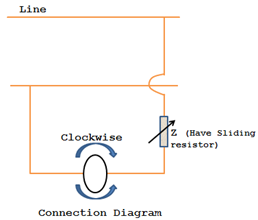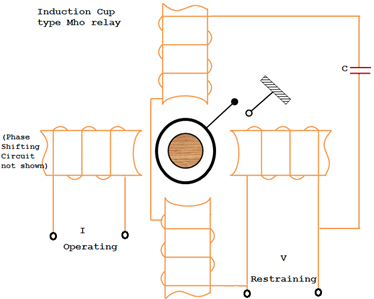The frequency of induced e.m.f. of synchronous generator is maintained constant by constant speed. Over speeding of the generator occurs due to loss of load and under speeding occurs due to increase in load. In both the cases, the frequency varies from normal value. In order to avoid damage to the generator under the above two conditions, frequency relays are used. Under frequency relay trips the feeder on load at set value of frequency, so as to give relief to the generator, thereby saving the unit. Under frequency relay thus aids load shedding programme to save the grid.

### Protective relay

The frequency relay is connected to the secondary of the V.T. The relay monitors the frequency continuously. It has two pairs of coils and is connected in parallel to the supply voltage through the impedance Z. The impedance will vary with frequency. Under normal conditions, a torque is applied on the cup of rotor due to change in impedance either in the clock wise direction or in the anti-clock wise direction, depending on the frequency is higher or lower than the desired frequency (rated frequency). The frequency setting is varied by the sliding resistor and the pickup can be varied by the restraining spring.

• Directional Over Current Relay

# Negative Sequence Relay

Negative sequence relays are used to protect electrical machines against overheating due to unbalance currents in stator. These unbalance currents cause heating of rotor and damage it. Unbalance three-phase currents have negative sequence components. These components rotate at synchronous speed in a direction opposite to the direction of rotation of rotor, including double frequency currents in the rotor.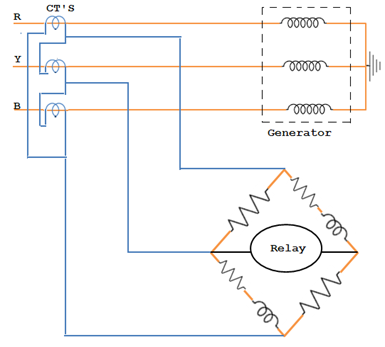The arrangement of negative sequence relay connection is shown in the figure. The relay is connected in parallel across the current transformer secondaries. Under normal conditions, as equal current flows in all the three phases, their algebraic sum is zero. Hence no current flows through the relay. But, if unbalancing occurs, the secondary currents will be different and the resultants current flows through the relay and the operation of the relay trips the circuit breaker to disconnect the generator from the system.

For unbalanced conditions or unsymmetrical faults, negative phase sequence network are used as shown in the figure below.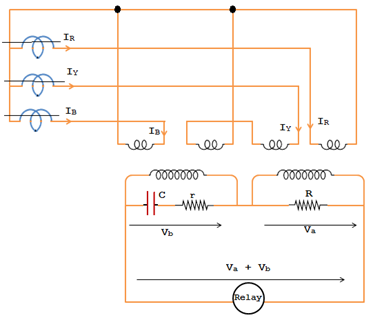• Types of Electromagnetic Relays

The values of c and r are such as to give a phase shift 60o. It can be seen from the vector diagrams that for the positive sequence currents the output voltage Va + Vb applied to the relay is zero shown in fig-a below where for the negative sequence currents, the output voltage Va + Vb is of considerable magnitude to operate the relay shown in fig-b.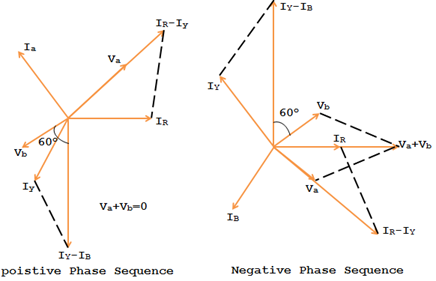The Negative Sequence Relay has the inverse square law characteristic. i.e., I22t = K, a constant. I2 is the negative sequence component of the current.

t = K / I22 i.e., t α 1/I22.

The relay will trip the generator main breaker.

This post first appeared on My Tech Info, please read the originial post: here

# Share the post

Under Frequency | Negative Sequence Relay

×